Next: 4.4 Numerical Implementation Up: 4.3 Advanced Illumination Aperture Previous: 4.3.1 Abbe's Method

## 4.3.2 Hopkins' Method

As already mentioned Hopkins' method is based on the exchange of the integration order over the point source contributions and the diffraction amplitudes, which has the advantage that a given optical system with fixed illumination, numerical aperture, defocus, and other aberrations can be described with the so-called transmission cross coefficients (TCCs). The TCCs need to be calculated just once. Thereafter they can be reused for repeated aerial image simulations of different mask patterns printed by the same optical system.

We will now derive the Hopkins' formulae only for the scalar aerial image calculation. The resulting relations are the discretized equivalents to those presented in . The extension to the vector case is obvious and can be found in the literature, e.g., in .

The aerial image Ii(x, y) is the incoherent superposition of the individual point source contributions Iipq(x, y) (cf. (4.83)). In the scalar theory these contributions are given by (4.54). With (4.51) and (4.52) the aerial image is explicitly written as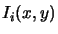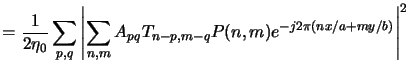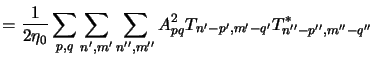(4.86)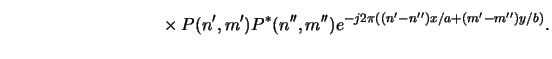Next we perform a change of summation indices like: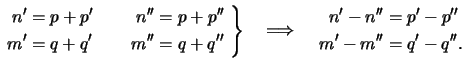(4.87)

By exchanging the summation order in (4.96) the aerial image is obtained as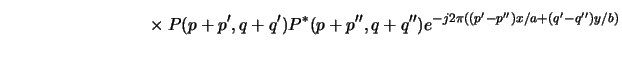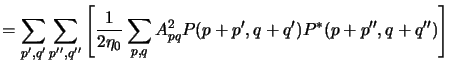(4.88)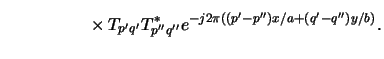The term in square brackets defines the TCCs, i.e.,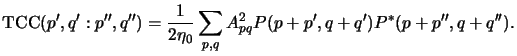(4.89)

The range of the various summation indices is illustrated in Figure 4.9 for the case of a circular-shaped illumination cone and pupil function. Hence, once the TCCs are calculated from (4.99) the aerial image can easily be determined for a fixed optical system from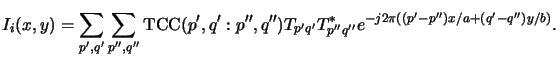(4.90)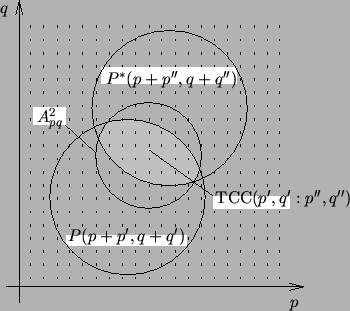Next: 4.4 Numerical Implementation Up: 4.3 Advanced Illumination Aperture Previous: 4.3.1 Abbe's Method
Heinrich Kirchauer, Institute for Microelectronics, TU Vienna
1998-04-17